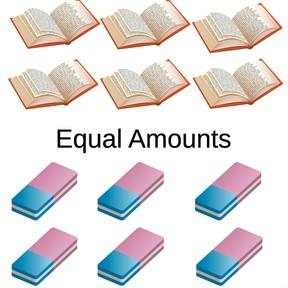Recognizing equal quantities 6-10

# Recognizing equal quantities 6-10

Recognizing equal quantities 6-10

No account needed.8,000 schools use Gynzy92,000 teachers use Gynzy1,600,000 students use Gynzy

## General

Students learn to recognize when things are in equal quantities from 6-10. They learn this by comparing different objects in different quantities with each other.

K.CC.C.6
K.CC.C.7

## Relevance

It is useful to know if you have more, less or equal quantities of something. An example is if you want to know if you have an equal quantity of marbles.

## Introduction

Count all of the balls together out loud. What kind of ball has the largest quantity? And the smallest quantity? Next check if students can count the different quantities of balls. You may choose to have the students can write down the answers. You can use the eraser or drag the covers to show the answer below.

## Development

Give one student 6 notebooks and another student 6 erasers. You can also choose different objects. Are these equal quantities? How do you know? Start by counting the first group out loud. Then count the second group out loud. Are the numbers the same? If so, they are equal quantities. Practice this by comparing boys vs girls to show the difference. Check if the students know what equal quantities are by dragging balls to the right side of the interactive whiteboard.

To check if students understand what equal quantities are, you can ask the following questions:
- What does equal quantities mean?
- Do you count equal quantities of erasers and dots?
- How do you know if something is in equal quantities?

## Guided Practice

Students practice by comparing different images with 6-10 objects and determine if they are equal quantities or not.

## Closing

Check if students have understood by playing to games. Drag an equal quantity of balls to the net and drag the fish from the aquarium to the container. Ask students how they can check that they have the right answer. They can count quantities a second time to see if they are and equal quantity.

## Teaching Tip

If students have difficulty, ask them to practice counting to 10 and by comparing quantities to 5. Students who are ready for more can be challenged to compare numbers 1-10 without images.

## Instruction materials

Notebooks, erasers

### The online teaching platform for interactive whiteboards and displays in schools

• Save time building lessons

• Manage the classroom more efficiently

• Increase student engagement